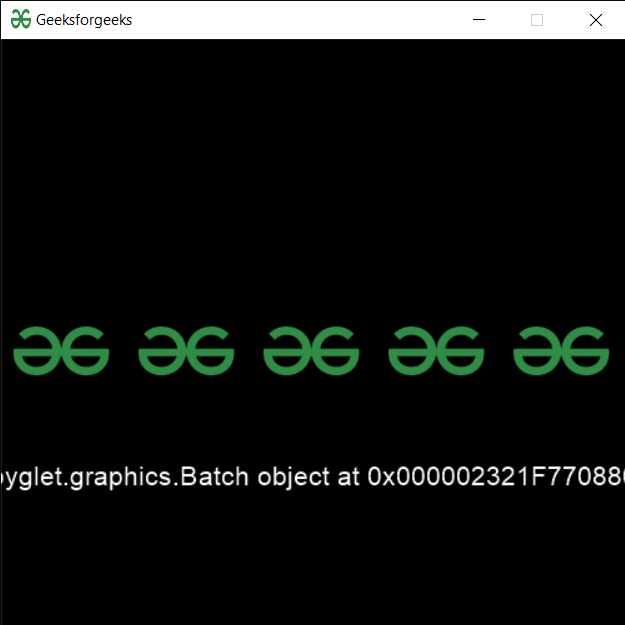Open in App
Not now

# PYGLET – Accessing Batch of Sprite

• Last Updated : 30 Sep, 2021

In this article we will see how we can access the batch object of sprite in PYGLET module in python. Pyglet is easy to use but powerful library for developing visually rich GUI applications like games, multimedia, etc. A window is a “heavyweight” object occupying operating system resources. Windows may appear as floating regions or can be set to fill an entire screen (fullscreen). A sprite is an instance of an image displayed on-screen. Multiple sprites can display the same image at different positions on the screen. Sprites can also be scaled larger or smaller, rotated at any angle, and drawn at a fractional opacity. Image is loaded with the help of image module of pyglet. The sprite can be migrated from one batch to another, or removed from its batch (for individual drawing). Note that this can be an expensive operation.

We can create a window and sprite object with the help of commands given below

```# creating a window
window = pyglet.window.Window(width, height, title)

# creating a sprite object
sprite = pyglet.sprite.Sprite(img, x, y)```

In order to create window we use batch attribute with sprite object
Syntax : sprite.batch
Argument : It takes no argument
Return : It returns batch object

Below is the implementation

## Python3

 `# importing pyglet module``import` `pyglet``import` `pyglet.window.key as key``   ` `# width of window``width ``=` `500``   ` `# height of window``height ``=` `500``   ` `# caption i.e title of the window``title ``=` `"Geeksforgeeks"``   ` `# creating a window``window ``=` `pyglet.window.Window(width, height, title)``   ` `# text ``text ``=` `"Welcome to GeeksforGeeks"``  ` `# creating label with following properties``# font = cooper``# position = 250, 150``# anchor position = center``label ``=` `pyglet.text.Label(text,``                          ``font_name ``=``'Cooper'``,``                          ``font_size ``=` `16``,``                          ``x ``=` `250``, ``                          ``y ``=` `150``,``                          ``anchor_x ``=``'center'``, ``                          ``anchor_y ``=``'center'``)`` ` ` ` `# creating a batch``batch ``=` `pyglet.graphics.Batch()`` ` `# loading geeksforgeeks image``image ``=` `pyglet.image.load(``'gfg.png'``)`` ` `# creating a list of sprites object``sprites ``=` `[]`` ` `# position of images``pos_x ``=` `10``pos_y ``=` `230`` ` `for` `i ``in` `range``(``5``):``     ` `    ``# temporary sprite object``    ``temp ``=` `pyglet.sprite.Sprite(image, pos_x, pos_y, batch ``=` `batch)``     ` `    ``# append the sprite object to the list``    ``sprites.append(temp)``     ` `    ``# increment the x co-ordinate``    ``pos_x ``=` `pos_x ``+` `100`` ` ` ` `# on draw event``@window``.event``def` `on_draw():``       ` `    ``# clear the window``    ``window.clear()``       ` `    ``# draw the label``    ``label.draw()``     ` `    ``# draw the batch``    ``batch.draw()``       ` `# key press event    ``@window``.event``def` `on_key_press(symbol, modifier):``   ` `    ``# key "C" get press``    ``if` `symbol ``=``=` `key.C:``         ` `        ``# printing the message``        ``print``(``"Key : C is pressed"``)``         ` `# image for icon``img ``=` `image ``=` `pyglet.resource.image(``"gfg.png"``)`` ` `# setting image as icon``window.set_icon(img)``    ` `    ` `# getting batch of the first sprite``sprite ``=` `sprites[``0``]``value ``=` `sprite.batch` `# setting this value to the label``label.text ``=` `str``(value)`  `# start running the application``pyglet.app.run()`

Output :My Personal Notes arrow_drop_up